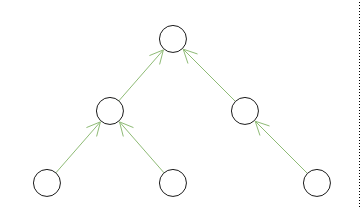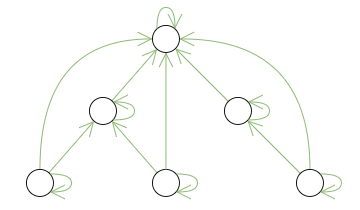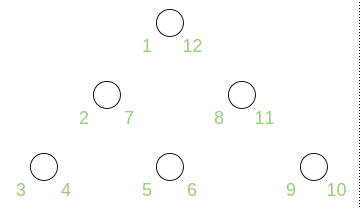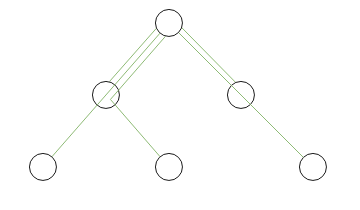• XML / Markup语法解析器（例如Apache Xerces和Xalan XSLt）使用树；
• PDF使用以下树结构：根节点->目录节点->页面节点->子页面节点。通常，PDF文件在内存中表示为平衡树。
• 多种科学和游戏应用程序使用决策树，概率树，行为树；Flare可视化库（http://flare.prefuse.org/）充分利用了生成树，经纪人在决定是否投标合同时也使用树木。

• 计算机科学中的二叉树，
• 生物学中的系统树
• 商业中的庞氏骗局，
• 项目管理中的任务分解树，
• 树用于建模多级关系，例如“上级-下级”，“祖先-后代”，“整个-部分”，“一般-特定”。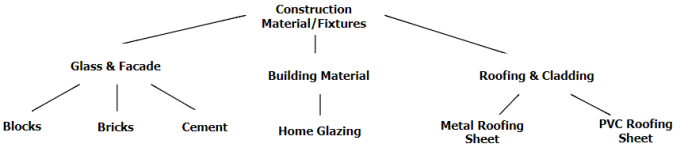## 1.邻接表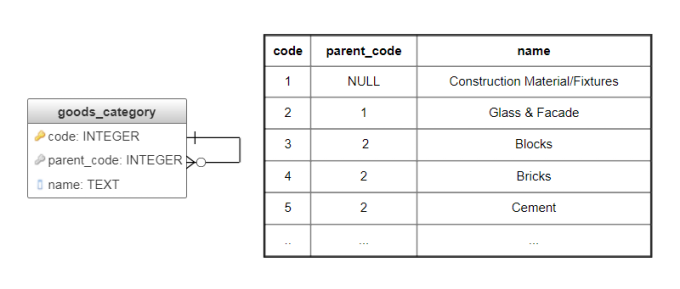``````WITH RECURSIVE sub_category(code, name, parent_code, level) AS (
SELECT code, name, parent_code, 1 FROM goods_category WHERE code=1 /* id of the given node */
UNION ALL
SELECT c.code, c.name, c.parent_code, level+1
FROM goods_category c, sub_category sc
WHERE c.parent_code = sc.code
)
SELECT code, name, parent_code, level FROM sub_category;
``````

``````WITH RECURSIVE sub_category(code, name, parent_code, level) AS (
SELECT code, name, parent_code, 1 FROM goods_category WHERE code=5 /* id of the given node */
UNION ALL
SELECT c.code, c.name, c.parent_code, level+1
FROM goods_category c, sub_category sc
WHERE c.code = sc.parent_code
)
SELECT code, name, parent_code, (SELECT max(level) FROM sub_category) - level AS distance FROM sub_category;
``````

``````WITH RECURSIVE sub_category(code, name, parent_code, level) AS (
SELECT code, name, parent_code, 1 FROM goods_category WHERE code=5 /* id of the checked node */
UNION ALL
SELECT c.code, c.name, c.parent_code, level+1
FROM goods_category c, sub_category sc
WHERE c.code = sc.parent_code
)
SELECT CASE WHEN exists(SELECT 1 FROM sub_category WHERE code=2 /* code of the subtree root */)
THEN 'true'
ELSE 'false'
END
``````

• 数据的直观表示（元组`(code, parent_code)`直接对应于树的边缘）；
• 没有数据冗余，也不需要非参照完整性约束（除非您需要DBMS级约束来防止循环）；
• 树木可以有任意深度；
• 快速，简单的插入，移动和删除操作，因为它们不会影响任何其他节点。

• 该方法需要递归以便选择节点的后代或祖先，定义节点的深度，并找出后代的数量。如果DBMS不支持特定功能，则无法有效地实现这些操作。对于无法进行递归或不可行的情况，可以通过使用一个递归来实现此方法的某些优点。

## 2.子集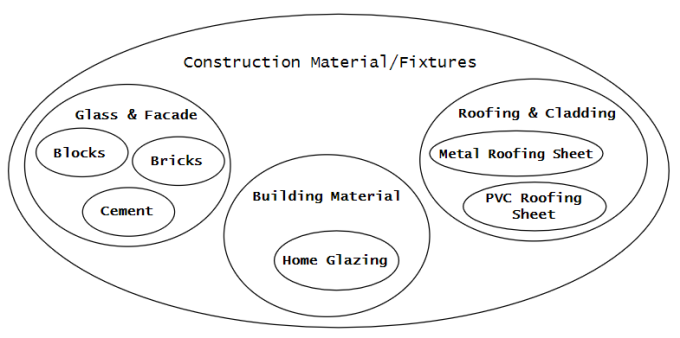Subsets方法需要两个表：第一个表包含所有子集（表`goods_category`，图4），第二个表包含子集的包含事实，以及相应的深度级别（表`subset`，图4）。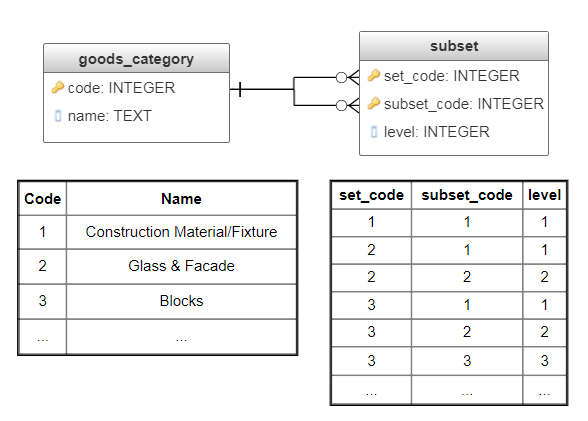``````SELECT name, set_code, subset_code, level FROM subset s
LEFT JOIN goods_category c ON c.code = s.set_code
WHERE subset_code = 1 /* the subtree root */
ORDER BY level;
``````

``````SELECT name, set_code, subset_code, level FROM subset s
LEFT JOIN goods_category c ON c.code = s.subset_code
WHERE set_code = 3 /* the give node */
ORDER BY level;
``````

``````SELECT CASE
WHEN exists(SELECT 1 FROM subset
WHERE set_code = 3 AND subset_code = 1)
THEN 'true'
ELSE 'false'
END
``````

Subsets方法的主要优点\是支持快速，简单，非递归的树操作：子树选择，祖先选择，节点深度级别的计算和后代数量。

• 相对不直观的数据表示（由于间接后代之间的冗余引用而变得复杂）；
• 数据冗余；
• 数据完整性需要触发器；
• 移动和插入操作比邻接列表方法更为复杂。插入需要为每个后代节点附加记录，而移动节点需要更新其后代和祖先的记录。

## 3.嵌套集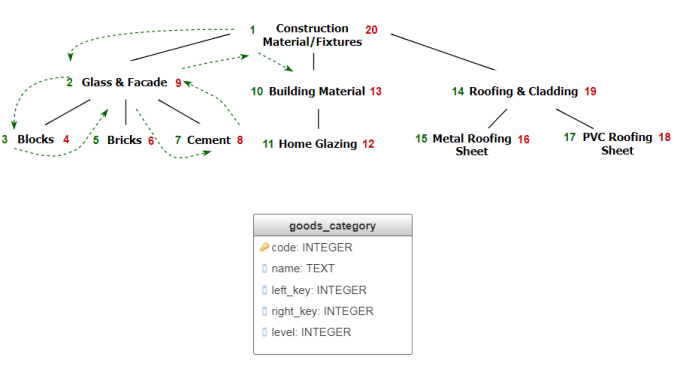``````WITH root AS (SELECT left_key, right_key FROM goods_category WHERE code=2 /* id of the node */)
SELECT * FROM goods_category
WHERE left_key >= (SELECT left_key FROM root)
AND right_key <= (SELECT right_key FROM root)
ORDER BY level;
``````

``````WITH node AS (SELECT left_key, right_key FROM goods_category WHERE code=8 /* id of the node */)
SELECT * FROM goods_category
WHERE left_key <= (SELECT left_key FROM node)
AND right_key >= (SELECT right_key FROM node)
ORDER BY level;
``````

``````SELECT CASE
WHEN exists(SELECT 1 from goods_category AS gc1, goods_category gc2
WHERE gc1.code = 1
AND gc2.code = 3
AND gc1.left_key <= gc2.left_key
AND gc1.right_key >= gc2.right_key)
THEN 'true'
ELSE 'false'
END
``````

## 4.物化路径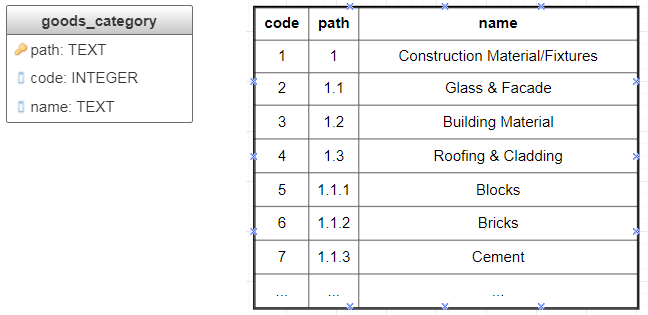``````SELECT * FROM goods_category WHERE path LIKE '1.1%' ORDER BY path
``````

``````SELECT * FROM goods_category WHERE '1.1.3' LIKE path||'%' ORDER BY path
``````

``````SELECT CASE
WHEN exists(SELECT 1 FROM goods_category AS gc1, goods_category AS gc2
WHERE gc1.name = 'Construction Material/Fixtures'
AND gc2.name = 'Cement'
AND gc2.path LIKE gc1.path||'%')
THEN 'true'
ELSE 'false'
END
``````

### 4.1。PostgreSQL的Ltree模块

PostgreSQL有一个指定的用于树的ltree模块：它允许以便捷的方式有效地使用Materializedpath方法。该模块实现一种`ltree`数据类型，用于表示存储在分层树状结构中的数据的标签。提供了用于搜索标签树的广泛工具。

``````CREATE EXTENSION "ltree";

CREATE TABLE goods_category_ltree (path ltree, name text);

INSERT INTO goods_category_ltree (path, name) VALUES
('construction_materials', 'Construction Material/Fixtures'),
('construction_materials.building_materials', 'Building Material'),
('construction_materials.glass.blocks', 'Blocks'),
('construction_materials.glass.bricks', 'Bricks'),
('construction_materials.glass.cement', 'Cement'),
('construction_materials.building_materials.home_glazing', 'Home Glazing'),
('construction_materials.roofing.metal_sheet', 'Metal Roofing Sheet'),
('construction_materials.roofing.pvc_sheet', 'PVC Roofing Sheet');
``````

``````SELECT * FROM goods_category_ltree WHERE path @> (SELECT path FROM goods_category_ltree WHERE name = 'Blocks');
``````

``````SELECT path, name FROM goods_category_ltree WHERE path ~ 'construction_materials.glass.*';
``````

``````SELECT * FROM goods_category_ltree WHERE path <@ (SELECT path FROM goods_category_ltree WHERE name = 'Construction Material/Fixtures');
``````

``````SELECT CASE WHEN exists(SELECT 1 FROM goods_category_ltree
WHERE (
SELECT path FROM goods_category_ltree WHERE name = 'Construction material/ Fixtures'
) @> (SELECT path FROM goods_category_ltree WHERE name = 'Cement'))
THEN 'true'
ELSE 'false'
END
``````

``````SELECT path, nlevel(path) FROM goods_category_ltree WHERE name ='Blocks';
``````

## 结论